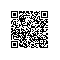# 初级排序算法1-定义排序规则

## 为什么学它

1. 对排序算法的分析将有助于你全面理解比较算法性能的方法
2. 类似的技术也能有效解决其他类型的问题
3. 排序算法常常是我们使用算法解决其他问题的第一步

## 排序算法类的模板

package algorithms4.sort;

import edu.princeton.cs.algs4.In;

public class Example {

public static void sort(Comparable[] a){
//排序算法
}

private static boolean less(Comparable v, Comparable w){
return v.compareTo(w) < 0;
}

private static void exch(Comparable[] a, int i, int j){
Comparable t = a[i];
a[i] = a[j];
a[j] = t;
}

private static void show(Comparable[] a){
//在单行中打印数组
for (int i = 0; i < a.length; i++) {
System.out.println(a[i] + " ");
}
System.out.println();
}

public static boolean isSorted(Comparable[] a){
//测试数组元素是否有序
for (int i = 0; i < a.length; i++) {
if (less(a[i], a[i -1])) {
return false;
}
}
return true;
}

public static void main(String[] args) {

//从标准输入读取字符串,将他们排序并输出
sort(a);
assert isSorted(a);
show(a);
}
}

## 性能评估

1. 运行时间.评估算法的性能,计算各个排序算法在不同的随机输入下的基本操作的次数(包括比较和交换,或者是读写数组的次数)

2. 在研究排序算法时,我们需要计算比较和交换的数量,对于不交换的算法,我们会计算访问数组的次数

3. 额外的内存使用

排序算法的额外内存开销和运行时间是同样重要的

排序算法可以分为两类

1. 除了函数调用所需的栈和固定数量的实例变量之外无须额外内存的原地排序算法
2. 以及需要额外内存来存储另一份数组副本的其他排序算法

## 数据类型

package algorithms4.sort;

import lombok.Data;

@Data
public class DateExample implements Comparable<DateExample> {
private final int month;
private final int day;
private final int year;

public DateExample(int d, int m, int y){
day = d;
month = m;
year = y;
}

@Override
public int compareTo(DateExample that) {
if (this.year < that.year) {
return -1;
} else if (this.year > that.year) {
return 1;
} else if (this.month < that.month) {
return -1;
} else if (this.month > that.month) {
return 1;
} else if (this.day < that.day) {
return -1;
} else {
return this.day > that.day ? 1 : 0;
}
}

@Override
public String toString(){
return year + "/" + month + "/" + day;
}

}



compareto必须实现一个完整的比较接口

1.自反性,对于所有的v,v=v

2.反对称性,有v<w情况的存在,都有v>w的情况存在

3.传递性,如果v<=w,且w<=x,则v<=x

compareto实现了我们的主键抽象,它给出了实现了comparable接口的任意数据类型的对象的大小顺序的定义使用钉钉扫一扫加入圈子
+ 订阅